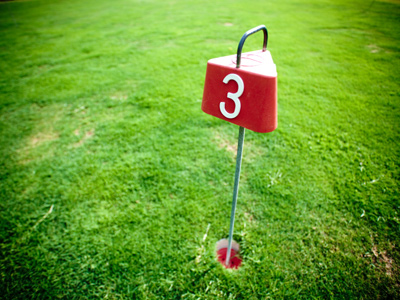A quarter of 12 is 3.

# Fractions (Year 3)

In KS2 Maths, kids will discover more about fractions. Starting in Year Three, they'll understand that a quarter is the same as 1 divided by 4, and a half is the same as two quarters.

Imagine sharing a cake with friends; you're dividing it into fractions. Fractions are numbers less than a whole. Do you know that one third is written as 1/3, and three quarters is 3/4? Can you figure out how many thirds or quarters make a whole? And which is bigger - two thirds or a half?

Practice what you've learned in Maths by playing this fun quiz all about fractions. Test your skills and see how good you are at recognising their values!

1.
Which of these is equivalent to 510?
12
23
34
14
If you divide 5 by 5 you get 1 and if you divide 10 by 5 you get two so 510 is the same as 12
2.
Which is the largest of these fractions?
12
510
23
24
12 is the same as 510 and 24
3.
One third and which other fraction make a whole one?
210
12
34
23
13 + 23 = 33 which is the same as 1
4.
Which is the smallest of these fractions?
12
14
13
110
If the numbers above the line are the same then the higher the number below the line the lower the fraction is
5.
What is a quarter of 12?
3
4
6
9
To calculate a quarter divide by 4
6.
Which fraction lies exactly between 12 and 1?
14
710
23
34
12 = 24 and 1 = 44 so 34 is between the two
7.
What does 110 mean?
One whole one divided into ten equal parts
Ten whole ones divided equally
Five whole ones divided equally
One whole one divided into two equal parts
110 is the same as 1 ÷ 10
8.
How many tenths in one whole?
5
10
2
4
A tenth is one part when the whole is divided into ten equal parts
9.
If we have 34 what is needed to make a whole?
12
13
14
110
34 plus 14 = 44 which is one whole
10.
Which represents one third?
14
12
13
15
13 is the same as 1 ÷ 3